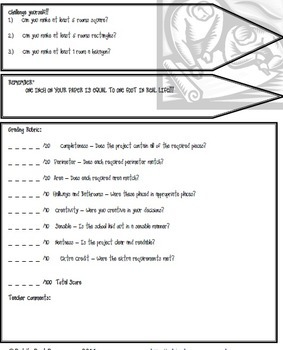# Bundle - Real Life Math Projects - Printable & Distance Learning Versions2nd - 6th, Homeschool
Subjects
Standards
Resource Type
Formats Included
•Google Drive™ folder
Pages
60 pages
\$29.00
Bundle
List Price:
\$33.00
You Save:
\$4.00
\$29.00
Bundle
List Price:
\$33.00
You Save:
\$4.00This bundle contains one or more resources with Google apps (e.g. docs, slides, etc.).

#### Products in this Bundle (7)

showing 1-5 of 7 products

### Description

Math is best learned in real life situations. This is a collection of 7 different math projects which put students into the real life situations and asks them to use their math. Each math project includes built in grading rubric to give students guidance and feedback. Working as individuals, or as groups, these projects are sure to get students' minds working. All 7 projects include a printable version AND a Google Classroom version.

This bundle includes all 7 of these projects:

the Ice Cream Shop Project which works on rounding, addition, subtraction, multiplication and division

the Be an Architect Project which works on area and perimeter, as well as creating a scaled drawing

the Holiday Shopping Project which works on addition, subtraction and rounding

the Party Planner Project which works on fractions (halving and quartering), doubling, tripling, addition, subtraction, multiplication, division and using money

the Balanced Checkbook Project which works on addition, subtraction, multiplication, division, rounding, budgeting and using money

the Holiday Recipe Project which works on measurement, rounding, addition and elpased time

the Field Trip Planning Project which works on elapsed time, budgeting, addition, subtraction, multiplication, division and using money

-------------------------------------------------------------------------------------------------------

Each project contains accurate spellings from the US and the UK. The projects cover a variety of standards including:

US Common Core Standards: 2.NBT.B.6, 2.NBT.B.7, 2.MD.C.8, 3.OA.A.3, 3.OA.D.8, 3.MD.C.5, 3.MD.C,6, 3.MD.C,7, 3.MD.D.8, 3.MD.A.1, 3.NBT.A.1, 3.NBT.A.2, 4.NBT.A.3, 4.NBT.B.4, 4.MD.A.3, , 4.OA.A.3, 4.MD.A.2, 5.NBT.A.4, 5.NF.B.4a, 5.NBT.B.6, 5.NBT.B.7

UK National Curriculum: Ma2.2.j, Ma2.3.h, Ma2.4.a, Ma3.4.e, Ma.2.3.e, Ma.2.3.g, Ma2.2.i, , Ma2.3.i, Ma2.3.j, Ma3.4.d

The Australian Curriculum: ACMNA075, ACMNA079, ACMNA080, ACMMG109, ACMNA053, ACMNA099, ACMNA059, ACMNA100, ACMNA101, ACMNA106, ACMNA105, ACMNA106, ACMNA128, ACMNA129, ACMMG086, ACMMG086, ACMNA076, ACMMG110

The Ontario Curriculum: Grade 3 Math - Measurement, Grade 3 Math - Number Sense and Numeration, Grade 4 Math - Measurement, Grade 4 Math - Number Sense and Numeration, Grade 5 Math - Number Sense and Numeration, Grade 6 Math - Number Sense and Numeration

The Revised National Curriculum of South Africa: Learning Outcome 1 - Numbers, Operations and Relationships - Grade 4, Learning Outcome 4 - Measurement - Grade 4, Learning Outcome 1 - Numbers, Operations and Relationships - Grade 5, Learning Outcome 1 - Numbers, Operations and Relationships - Grade 6

-------------------------------------------------------------------------------------------------------

Created by Heidi Raki of Raki's Rad Resources.

Total Pages
60 pages
Rubric only
Teaching Duration
2 months
Report this Resource to TpT
Reported resources will be reviewed by our team. Report this resource to let us know if this resource violates TpT’s content guidelines.

### Standards

to see state-specific standards (only available in the US).
Interpret the product (𝘢/𝘣) × 𝘲 as a parts of a partition of 𝘲 into 𝘣 equal parts; equivalently, as the result of a sequence of operations 𝘢 × 𝘲 ÷ 𝘣. For example, use a visual fraction model to show (2/3) × 4 = 8/3, and create a story context for this equation. Do the same with (2/3) × (4/5) = 8/15. (In general, (𝘢/𝘣) × (𝘤/𝘥) = 𝘢𝘤/𝘣𝘥.)
Solve multistep word problems posed with whole numbers and having whole-number answers using the four operations, including problems in which remainders must be interpreted. Represent these problems using equations with a letter standing for the unknown quantity. Assess the reasonableness of answers using mental computation and estimation strategies including rounding.
Solve two-step word problems using the four operations. Represent these problems using equations with a letter standing for the unknown quantity. Assess the reasonableness of answers using mental computation and estimation strategies including rounding.
Use multiplication and division within 100 to solve word problems in situations involving equal groups, arrays, and measurement quantities, e.g., by using drawings and equations with a symbol for the unknown number to represent the problem.
Add, subtract, multiply, and divide decimals to hundredths, using concrete models or drawings and strategies based on place value, properties of operations, and/or the relationship between addition and subtraction; relate the strategy to a written method and explain the reasoning used.